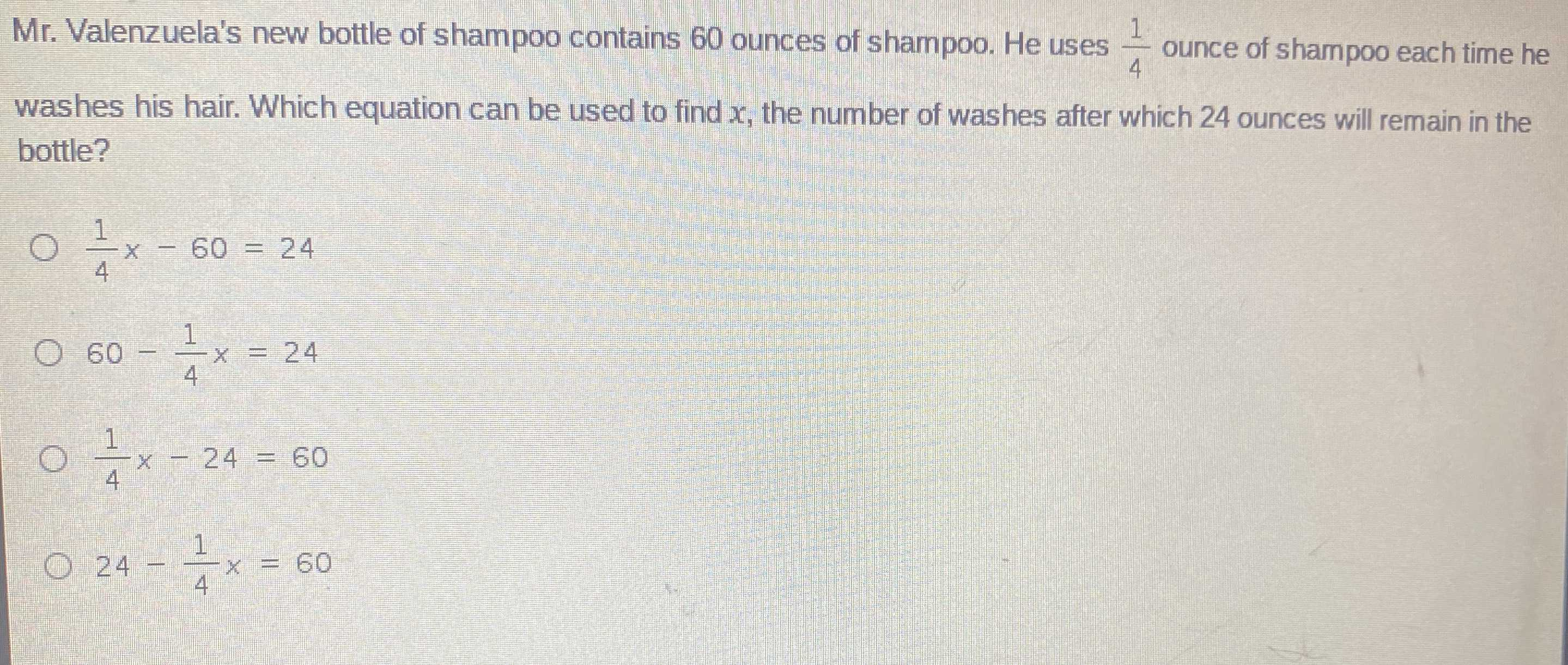### Still have math questions?

Algebra
QuestionMr. Valenzuela's new bottle of shampoo contains $$60$$ ounces of shampoo. He uses $$\frac { 1 } { 4 }$$ ounce of shampoo each time he washes his hair. Which equation can be used to find $$x$$ , the number of washes after which $$24$$ ounces will remain in the bottle?

$$\frac { 1 } { 4 } x - 60 = 24$$

$$60 - \frac { 1 } { 4 } x = 24$$

$$\frac { 1 } { 4 } x - 24 = 60$$

$$24 - \frac { 1 } { 4 } x = 60$$